### Home > CALC > Chapter Ch10 > Lesson 10.2.2 > Problem10-108

10-108.
1. Examine the integrals below. Consider the multiple tools available for integrating and use the best strategy. After evaluating each integral, write a short description of your method. Homework Help ✎

1.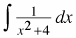2.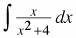3.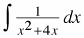4.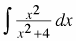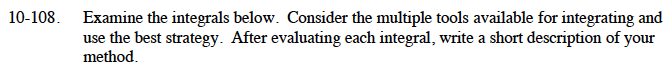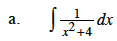You should recognize the integrand as a form of the derivative of tan–1(x).
The denomintor needs to be of the form a2 + 1.

$\frac{1}{x^2+4}=\frac{1}{4(x^2/4+1)}=\frac{1}{4((x/2)^2+1)}$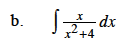Use substitution. Let u = x2 + 4.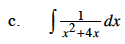Factor the denominator then use partial fraction decomposition.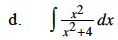$\frac{x^2}{x^2+4}=\frac{x^2+4-4}{x^2+4}=1-\frac{4}{x^2+4}$